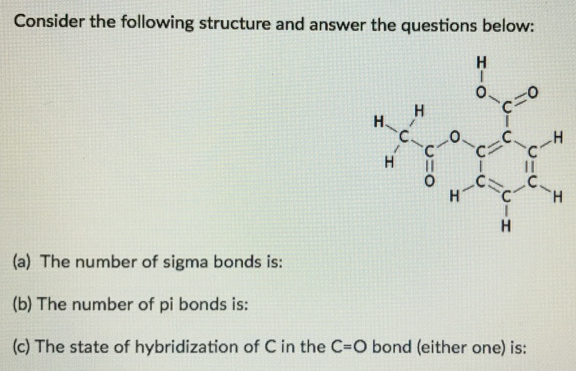# Problem: Consider the following structure and answer the questions below:a. The number of sigma bonds is:b. The number of pi bonds is:c. The state of hybridization of C in the C=O bond (either one) is:

###### FREE Expert Solution###### Problem Details

Consider the following structure and answer the questions below:

a. The number of sigma bonds is:

b. The number of pi bonds is:

c. The state of hybridization of C in the C=O bond (either one) is: